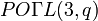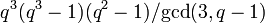# Endomorphism structure of projective special linear group of degree three over a finite field

## Contents

This article gives specific information, namely, endomorphism structure, about a family of groups, namely: projective special linear group of degree three.
View endomorphism structure of group families | View other specific information about projective special linear group of degree three

### Automorphism structure

For any prime power$q$, the automorphism group of the projective special linear group of degree three$PSL(3,q)$ over the finite field$\mathbb{F}_q$ is the projective outer semilinear group of degree three$PO\Gamma L(3,q)$.

Let$q = p^r$ where$p$ is the underlying prime. The information is presented below:

Construct Value Order Comment
automorphism group projective outer semilinear group of degree three$PO\Gamma L(3,q)$$2rq^3(q^3 - 1)(q^2 - 1)$ When$r = 1$, this is the same as the projective outer linear group of degree three.
inner automorphism group projective special linear group of degree three$PSL(3,q)$$q^3(q^3 - 1)(q^2 - 1)/\operatorname{gcd}(3,q-1)$ The group is identified with its inner automorphism group because it is a centerless group.
outer automorphism group
Case$q$ is not 1 mod 3: direct product of cyclic group of order 2 and cyclic group of order$r$
Case$q$ is 1 mod 3: Direct product of cyclic group of order 6 and cyclic group of order$r$$2r \operatorname{gcd}(3, q - 1)$
Case$q$ is not 1 mod 3:$2r$
Case$q$ is 1 mod 3:$6r$

### Other endomorphisms

Projective special linear group is simple, and in particular$PSL(3,q)$ is always simple, so the only endomorphisms are the automorphisms and the trivial endomorphism.